THE SPACE ELEVATOR
- PHYSICAL PRINCIPLES -

Patent No. 02-1712-29122000 HAA

INTRODUCTION

Be serious and don't be surprised at the following question:

Ý Have you ever seen the rope hanging from the space? Or, better to say the rope which end disappears in the azure of the sky? Yes, you answer, but only in the cartoon about Aladdin. In reality - never! If you saw in reality, or if you saw somebody climbing up that rope, you wouldn't believe your own eyes. Your common sense tells you that the rope should be hooked up on something.

However, believe me, I'll exactly prove in this article: the rope that hangs from the sky is absolutely possible and that without any conjuring tricks. I must be more precise and it is better to say like this: the rope is fastened on the surface of the Earth, consequently on the ground, and it falls into the sky.

To achieve that, we must have very, very long rope. With regard to the development of new materials, the matter becomes practicable in the near future.

Ý How did I get this idea? In the year 1998 I was driving from Zadar across the Velebit pass to Zagreb. On the right side of the road, I saw huge gray rocks over which the long distance power lines were placed. The azure of the sky, the grayness of the rocks and the lines stimulated me to think. How would it be that the lines stand vertically...? Nonsense... So it all started. Wandering in my "thought experiment", I had this interesting idea. I have described quantitatively important things of this problem by physical approach and by equations. The results and the possible applications are astonishing. However, I was even more surprised when I realized that other authors were also working on the same topic [1-9] . The results that I have obtained persuaded me in correctness of the physical way, and that I am not the only one who is thinking about the space topics.

Geostationary satellites are the bodies that rotate in the equatorial plane around the Earth on the height of 35790 km above the ground. As they rotate with the same angular speed as the Earth, looking from the Earth they seem to be immovable. In this article a very long, lengthened geostationary satellite - cable that touches the surface of the Earth is described.

THE CABLE OF GREAT LENGTH ON THE SYNCHRONOUS AXIS

Ý Imagine the point A, which is at standstill on the ground on the Earth equator. Imagine the radial straight line from the center of the Earth through the point A. As the point A is at standstill, this straight line would rotate together with the Earth with the same angular speed as the angular speed of the Earth. Therefore, in the further text this straight line is called the synchronous axis, and the point is called the hanging point.

Now, let's make a thought experiment. We put a very long cable alongside the synchronous axis, which is fastened on the Earth on the point A.

The gravitational force influences on each particle of the mass of that cable, and it declines with the square distance from the Earth. On account of the rotation of the synchronous axis, the centrifugal force appears. This force grows linearly with the length of the cable. Both forces influence alongside the synchronous axis, but in the opposite directions. Which force will prevail depends on the length of the cable.

Ý Suppose that the cable is of extremely huge length. In that case the centrifugal force prevails. On account of two opposite forces, the cable is tight along its all length. Looking from the point A, the cable is statically in a balanced manner and it stands vertically.

The main request for the cable

These two conditions must be absolutely realized:

• The stress must be constant and equal on any point of the cable
• The stress must be less than the critical stress

Ý The effect of that is that the thickness is changing as we move alongside the cable. In that way the cable is equally loaded on each point and it will not break anywhere.

The following picture demonstrates the cable element:F – tension force (N) x – cable radius (m) D x – radius changing (m) D y – cable length element (m) r - cable density (kg/m3) D V – cable element volume (m3) p – stress (N/m2) -

The upper request for the stress can be mathematically expressed in the following form:

Ý It is easy to show that the volume of the cable element (Figure 1) is determined with this expression:

The function g(r) in the formula (1) is acceleration that influences on the cable element. It is changed with the distance from the Earth center in the following way:

Ý The first member on the right side is gravitational attraction of the Earth, and the second member is centrifugal acceleration on account of the Earth rotation. The third member includes the gravitational influence of the Moon. In the case when the Moon is in the line above the hanging point, we take the upper sign, and in the case when the Moon is in the line below the hanging point, we take the lower sign.

In the same way as for the Moon, it is possible to add the fourth member as well, which will include the gravitational influence of the Sun too. On account of the weaker influence of the Sun, that member is omitted.

The parameters in the formula (3) are:

 M = 5.976*1024 kg the Earth mass g = 6.6732*10-11Nm2/kg2 general gravitational constant w 0 = 7.2921*10-5s-1 the Earth angular speed Mm= 7.35*1022 kg the Moon mass rm= 384.4*106 m the distance from the Earth to the Moon R = 6.378*106m the Earth radius y the distance from the ground (altitude)

Ý The distance from the Earth center is:

By inserting the relation (2) in (1) we get the following equation:

Suppose Dx ® 0. Taking into consideration (4) we get the following differential equation:

which after the integration gives the solution:

Ý The gravitational influence of the Moon will influence very weakly on the cable profile, so that member in the upper equation can be neglected. So, the equation assumes simpler form:

where k(r) is of this form:

After inserting (4) in (8) we get the formula for the cable radius:

Ý This formula completely satisfies the condition expressed by the equation (1).

For example, we have the cable with the ratio r / p = 4.7619*10-8 s2m-2. Its initial thickness is d = 2x0 = 1cm. In the further text that cable is called super-cable. What is its profile like?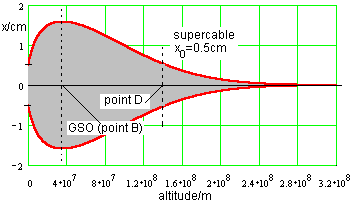Figure 2. What a wonderful shape! On the thickest point the cable is 3 times thicker than on the ground. There is a question on the cable profile made of the material that is known to us? That cable would have a huge stomach. That is the topic in the further text.

Where is the "stomach" of the cable?

Ý The cable has its maximum thickness in the point:

That is the point that is situated 35790 km above the equator. Consequently, the "stomach" of the cable is just on the geostationary circle. The total cable is one very elongated geostationary satellite, which extends to the ground!

Ý In the formula (10) the parameters p and r do not appear. The conclusion follows: no matter of what material the cable is made, the point of the maximum thickness is always situated on the geostationary circle. The position of the "stomach" or the geostationary circle depends on the mass, the radius and the rotation speed of the celestial body.

The formula (10) is inserted in (9) and we get:

The value of K (for the Earth) is 4.8448*107 J/kg. The point of the maximum thickness, which is 35 790 km far from the ground, is called the "point B".

Ý Looking at the cable profile on the previous figure, we can notice that the thickness is growing at first, and then it diminishes longing for zero. Find the point y where the cable thickness is the same as on the ground, or mathematically: x(yD) = x0. It is easy to find out that the value yD = 1.438*108m satisfies that condition.

That point, which is 143 800 km far from the ground, is called the "point D".

Under the term the cable length we consider the length where the cable is of the same thickness on the both ends. Consequently, the cable length is 143 800 km (although it can be in reality shorter or longer).

Ý According to the formulae (9) and (11), the cable thickness depends on the ratio of density and the stress. The effect is physically interesting as that ratio appears in the exponential function. The consequence of that is that the profile, the volume and the total mass of the cable are extremely sensitive on the ratio r/p. For the building of the cable we need materials where that ratio is as less as possible.

Let's make the estimate for the Earth and the Mars. The parameters for the Mars are as follows:

 R = 3.397*106 m the Mars radius T = 8.866*104 sec. rotation time round its own axis w 0 = 7.086*10-5 sec-1 angular speed round its own axis M = 6.47*1023 kg the Mars mass yB = 1.71*107 m "Mars-stationary" circle (see the formula (10)) yD = 6.606*107 m the point D K = 9.572*106 J/kg the constant K (see the formula (12))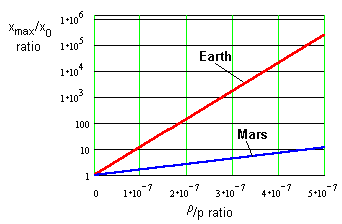Figure 3. On the figure the facts which the formula (11) gives are shown for the Earth and the Mars. As the value of the constant K in the formula (12) is smaller, the requests for the materials in the case of the Mars are not so strict. The building on the Mars would be possible even from the materials that we already have (see the following table). Notice that the vertical axis is logarithmic.

Ý The following table shows the ratio of the maximum and initial cable thickness for some materials (the facts are shown according to the formula (11) for the Earth and the Mars):

 SUBSTANCE DENSITY (kg /m3) TENSILE STRENGTH (N/m2) r /p RATIO (s2/m2) xmax/x0 ratio EARTH xmax/x0 ratio MARS TRANSVERSE WAVE SPEED (m/s) fused silica 2.2*103 ? _ _ _ _ pyrex glass 2.32*103 ? - _ _ _ steel 7.9*103 (1-5)*109 (1.58-7.9)*10-6 4.2*1016-1.3*1083 1920-2.63*1016 _ E-glass fiber 2.54*103 2.4*109 1.068*10-6 1.72*1011 166 968 carbon fiber 1.85*103 (2-5)*109 (3.7-9.25)*10-7 7810-5.39*109 5.9-83.7 _ boron fiber 2.45*103 3.5*109 7*10-7 2313000 28.5 1195 berylium fiber 1.87*103 3.3*109 5.67*10-7 922700 15.1 1328 S-glass fiber 2.49*103 4.5*109 5.53*10-7 657300 14.1 1345 Kevlar 49 (armid fiber) 1.44*103 3.6*109 4*10-7 16150 6.8 1581 Spectra 1000 fiber 0.97*103 3.0*109 3.23*10-7 2501 4.7 1760 Spectra 2000 fiber 0.97*103 3.51*109 2.76*10-7 801 3.75 1903 Zylon (PBO, plastic fiber) 1.57*103 5.8*109 2.71*10-7 710 3.66 1921 buckytube cable (theoretical data) 1.3*103 150*109 8.67*10-9 1.2 1.04 10740

Table 1.

Ý From the upper table it is evident that the use if steel for the building of the cable on the Earth is really absurd. Other materials are also useless, because they give too thick profiles, which would have huge mass, thanks to the great length of the cable.

The only hope is nanotubes ( buckytube cable ), where that ratio is exceptionally convenient for the building of the cable. However, longer macroscopic samples have not been made up to now, and the nanotubes have been produced in microscopic quantities. Due to intensive researches and development on that field, there is a great possibility that such a material will be available in the near future.

It is carbon that is very much represented for the building of the cable in the Universe, so it shouldn't be lifted up from the Earth. The production of the pure crystals in the high vacuum and the conditions of the smaller gravitation would have great advantages.

Ý Looking from the point A, a complete cable that lies on the synchronous axis, is statically balanced.

The length of the cable can be shortened by putting the compensate mass mK on the other end of the cable. That mass compensates the rest of the cable and it must satisfy the following formula:

y is the cable length and x is the cable radius on its other end.

Ý For all that, the condition that y > yB must be fulfilled. The cable must be so long to pass behind the geostationary circle.Figure 4.   The figure shows the facts for the super-cable with the initial thickness of 1 cm. The compensate mass diminishes very quickly with the distance from the equator. Notice that the vertical scale is logarithmic.

Ý It is interesting to mention that the Mars satellite Deimos is 20000 km far from the surface of the Mars, respectively about 3 000 km behind the Mars geostationary circle. The plane of the rotation is declined for about 20 from the Mars equatorial plane. It would be possible to take material for the compensate mass from the Deimos.

But, there is an obstruction! Another (greater) Mars satellite the Phobos rotates much closer to the Mars, that is on the height of 5980 km above the Mars ground and the plane rotation is declined for about 10. In specific time intervals the Phobos would strike against the cable. It is possible to solve even this problem, but that is not the topic of this article.

THE SPACE ELEVATOR

Ý The exhibited physical principle imposes the following possible application: a long cable which lies on the synchronous axis could be used as the "line" on which, in both directions, useful load could move on the great distances. It would be the "bridge to the stars" or the space elevator, which could have great advantages in the relation to the classical rockets.

How to determine the weight and the mass of useful load, which could move from the surface of the Earth up by the cable? It is easy to answer to that question: Maximum weight GMAX of useful load must satisfy this relation:

but naturally, the condition p < pcrit. must be fulfilled. Then the mass of useful load is:

From the relation (3) it follows that g(r) diminishes as the distance from the ground increases. Therefore on the higher heights it is possible to lift loads with greater mass.

Ý Because of the movement of the load, Coriolis force influences on it and this force endeavors to throw out the whole system from the synchronous axis. As the mass of the complete elevator is much greater than the mass of the load, and the cable over all its length is tight, Coriolis effect is nearly negligible. Little oscillations of the elevator round the synchronous axis, which could appear, can be eliminated by the changing of the speed movement of the load alongside the cable. That very long centrifugal pendulum is the topic for another article. However, it is important to say that the influence of Coriolis force wouldn't cause to topple the project of the space elevator.

For example, we have the super-cable on the Earth with the initial thickness of 1 cm. The formula (14) gives the maximum mass of the load of 168 tones, that could be lifted up from the ground by the super-cable.

The load of maximum 1200 tons mass could be lifted up from the ground by the use of the cable with the same initial thickness, which is made from the nanotubes.

Ý Even from the surface of the Mars the same cable could lift the load of greater mass up, because the Mars gravitation is 2.65 times weaker than the Earth gravitation.

It would be very interesting to estimate total mass of this very long cable. The elementary cable volume is estimated on the following way:

x(r) and k(r) are defined by the formulae (8) and (8a). The total volume is found out by the numerical integration.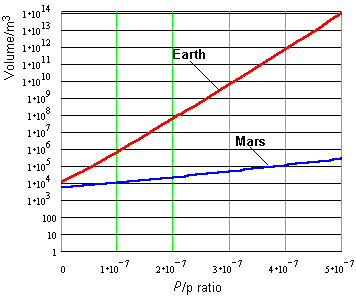Figure 5.   The volume dependence of the cable on sorts of materials (logarithmic tables). The cable on the Earth is 143 800 km long, and on the Mars it is 66 060 km long (the "point D") The initial cable thickness is 1 cm. As we can see, the total volume (and mass) is extremely sensitive on the ratio of density and the stress.

Gravitational influence of the Moon

Ý The Moon influences on the cable and on the compensate mass. Therefore, additional force appears on the hanging point on the ground, which tends to tighten the cable even more or to loosen it, depending on the side where the Moon is. This is similar to the phenomenon of high and low tide on the sea. Additional force, which tightens the cable when the Moon is above the hanging point, is: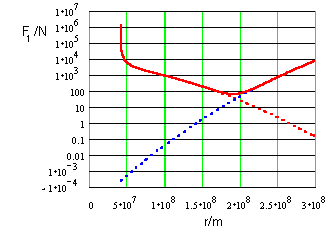Figure 6.  The figure shows the gravitational influence of the Moon when it is above the hanging point. For the specific value of r (the point where there is the compensate mass) there is minimum of the function F1 (r). The influence of the Moon is weakly expressed.

Ý In the case when the Moon is below the hanging point, the force that tends to loosen the cable is even weaker, and we can estimate it by the use of the following formula:

Comparing the powers F1 and F2 with the power Gmax (the cable tension in the hanging point), we can notice that the tidal obstruction of the Moon is very weak and it can't significantly influence on the cable stability. That particularly concerns the value of r, for which the function F(r) has minimum. Similar conclusion is also for the tidal power of the Sun.

Transverse waves

Ý On account of the possible influence on the construction stability, which is placed completely on the synchronous axis, it is necessary to describe the expansion of the transverse wave alongside the cable. The cable mass on the length unit is:and the cable tension is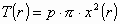Taking into consideration these two formulae, we find out that the speed of transverse wave expansion is:

Although the cable thickness is changeable, the transverse waves are expanded by the constant speed!

Ý As the body is climbing up alongside the cable, its kinetic energy is growing. On the certain point of the cable, the body achieves the escape velocity. In that case the sum total of the kinetic and potential energy is equal to zero:

from where we get that rC = 5.314*107 m, respectively yC = rC – R = 46 770 km. The point yC on the cable is named the "point C". Separated from the cable on the point y > yC, the body becomes free.

Ý The point on the synchronous axis y = yB is placed on the geostationary circle. On that point the gravitational and centrifugal powers are equaled.

On the distances y < yB the gravitational attraction overcomes, therefore the body slides toward the Earth. If it is y> yB, the centrifugal power overcomes therefore the body removes from the Earth, sliding over the synchronous axis.

The point y = yB on the geostationary circle is balanced position, the point where the bodies can slide on one or the other side of the synchronous axis.

In any case, the total geostationary circle is one privileged place for the future. If the body would separate from the cable, the Kepler's laws determine its further motion and fate.

Ý From the above exposure it follows that we must spend energy only when the body is in motion from the ground to the geostationary circle, that is to the point on the synchronous axis where y = yB. After that point it is not necessary any more to invest energy. The body itself (without any operation) accelerates removing from the Earth. So we can get energy by slowing down that body! Instead of thermal, we could get electrical energy.

We make the energetic calculation on the following way:

Let's the load mass m removes from the surface of the Earth alongside the synchronous axis. The motion is in the direction of the centrifugal force, so that force has done the following work:

Work of the gravitational force is negative because the force and the shift are in the opposite directions:

Therefore, for lifting up 1 kg of load on the height y alongside the synchronous axis, it is necessary to invest the work:

It is interesting to mention that the same expression appears in the exponent from (9).Figure 7. The graph of the function (23) for the Earth and the Mars. The maximums are placed on the geostationary circle. Lifting the load on the greater distances are energetically more profitable. Zero point on the graph is the point on the synchronous axis, where we can lift up the load without energy expense. Everything is done on account of the rotational energy of the Earth! The point is named the "point D". Behind that point we could produce energy!

Ý Looking energetically, the cable enables driving free of charge! To compare, to launch the load by the rocket, we must invest minimum energy of 62.5 MJ/kg or 17.36 KWh/kg. That is the energy necessary to loosen from the Earth.

 EARTH MARS altitude/km total energy/MJ/kg altitude/km total energy/MJ/kg point A 0 0 0 0 point B 35 810 48.56 17 090 9.572 point C 46 770 47.70 22 430 9.387 point D 143 910 0.00 66 060 0.00

Table 2.

Besides that the point D is energetically interesting, we have to point out that on this point (according to (9)), the cable is of the same thickness as on the ground.

Ý In the physical sense the cable of smaller length would be quite possible, and it is so called the "levitating cable". The complete system would be a thin, very long geostationary satellite. On the both ends there are compensate masses m1 and m2. The cable wouldn't touch the Earth, but it could arbitrarily come closer with one of its ends.

Nearly the same considerations will be valid as with the inference of the equation (9). If the cable radius on its closer end is x0, then the radius on any point is:

The compensate masses on the both ends must be:

The parameters a and b are the mass distances m1 and m2 from the Earth center.

Smaller ratio r/p enables greater cable length, that is the satellite can come closer to the ground. Anyway, these requests, on account of smaller length, are not so strict comparing with the cable that touches the Earth.

Ý This system does not use the Earth rotational energy, and it wouldn't be suitable in the function of lifting the load. It would be low altitude geostationary satellite, which the basic purpose would be improving telecommunications by the use of lower power of transmitters.

The upper physical principle would be valid also for the other very longish satellites, which are not geostationary. Such a rotating systems would have complicated dynamics, and they would request adding of energy from the outside and are not physically interesting.

Ý According to the previously displayed physical principles, the cable which is fixed by one of its ends for the Moon's ground, would be possible. The other end of the cable would be constantly directed toward the Earth, but it wouldn't touch it. Acceleration, which influences on the cable element, is:

With the same process as for the inference of the equation (9), we get the cable radius: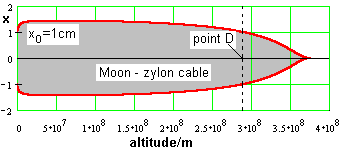Figure 8. The cable profile, which is fixed on the surface of the Moon and constantly directed toward the Earth. The point D of the cable is on the height of 290 100 km above the Moon's ground. The cable does not have strong requests concerning the materials. The compensate mass would be great because of the Earth's nearness. It is interesting to mention that because of this cable, the eccentricity of the Moon's orbit round the Earth would become a little greater.

The cable profile from the other side of the Moon is described by the following equation:

The cable would have tremendous length of 679 769 km (the point D), but it could be very thin.

Ý The compensate mass (the artificial satellite) would be great. From any point on that cable, which is on the height y>142500 km, the Earth would be seen. That would enable communication between the Earth and the other side of the Moon. The parameters in the formulae (27), (28) and (29) are:

 Rm = 1.739*106m - the Moon radius w = 2.6617*10-6 s-1 - the Moon angular speed d = 4.6738*106m r0 = 3.8226*108m r1 = 3.8614*108m -

BUILDING OF THE ELEVATOR

Ý The most demanding task in the technical sense would be the building and the setting up the first cable on the synchronous axis. The idea is as follows:

The building would always begin from the geostationary circle. First, the "stomach" of the future elevator would be built, and then simultaneously it would be built from the both sides of the "stomach". At the same time, the following three conditions must be strictly satisfied:

1. The complete system in the building, in any moment, must be the geostationary satellite

2. The complete system in the building, in any moment, must lie on the synchronous axis

3. During the building the cable thickness on the both ends must be equal, although it is constantly changing

During the building the system is the "levitating" cable (24), until it reaches the Earth. The compensate masses m1 and m2 are in fact the mobile building-sites, which are constantly moving apart while the cable is building. The material for the cable is delivered on the geostationary circle, and then alongside the cable in two opposite directions.

The cable length during the building or the distance between the m1 and m2 must satisfy in any moment, because of the condition No. 3, the following relation: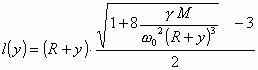(29)

y is the mass distance m1 from the ground ( the first mobile building-site ).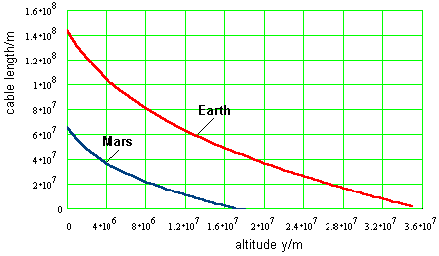Figure 9.  The building of the cable begins from the point y = yB = 35810 km (for the Earth) and from the point y = 17090 km (for the Mars). As it is shown on the graph, the cable length is then equal to zero.

Ý During the building, the compensate masses at the ends of the cable may have arbitrary value. Anyway, to fulfill the condition No.1, the ratio of those masses in any moment must be: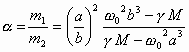(30)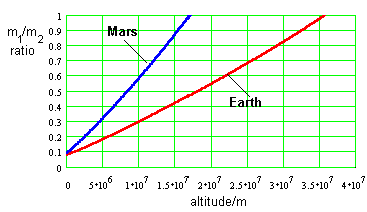Figure 10. On the figure the ratio m1/m2 is shown during the building for the Earth and the Mars as the function of distance for the first mobile building-site m1 from the ground. It can be seen from the graph that m1

Ý During the building under the influence of Coriolis force the total system would twist for a certain angle in the equatorial plane, so the cable would cut the synchronous axis in the point on the geostationary circle. The necessary momentum for canceling this effect could be achieved by small side correcting forces on each end of the cable. So still the condition No. 2 would be satisfied.

CONCLUSION

Ý The basic application of the equation (9) would be the building of the space elevator. The most important thing for realizing such a world project is (cheap) material production, where the ratio r /p is as less as possible. That material must also satisfy other very high requests.

Looking energetically, the space elevator is the device that uses the Earth's rotational energy. Lifting up the load on greater distances is energetically very profitable. As the difference from the rocket, lifting up and landing can be done continuously. The price for drive would be law. That is the only way for massive lifting up of the load in the future.

Other uses are possible, as for example the transmission of the electric power to the ground, improvement of the telecommunications etc. The space elevator would be ecologically very acceptable. All these are facts that make it superior in relation with the classical rockets.

Ý The greatest barrier in the technical sense and the most demanding task would be lifting up and setting up the first cable on the synchronous axis. Other systems that would be in a great number on the equator would be built by means of one other. Such a systems or "space highways" would be radial arranged round the equator like the pricks of a hedgehog. It would be the beginning of the geostationary circle building as the object or another man's home. That "object" would be important intermediate stop for travelling into the space.

Physical principles also enable the building of the so called the "levitating cable". Such a geostationary satellite would be much closer to the ground than the other geostationary satellites.

Today, while I am revising this article for the Internet, it is Christmas, 25th December 2000. The project of the space elevator is too much ambitious for this millennium. For the next it surely isn't. Therefore, I have to be patient still for a few days!

Ý REFERENCES:

 John D. Isaacs, Allyn C. Vine, Hugh Bradner and George E. Bachus, Satellite elongation into a true "Sky-Hook", Science 151, 682-683 (1966).

 Vladimir Lvov "Sky-Hook: Old Idea," Science, Vol. 158, November 17, 1967, pp. 946-947.

 Jerome Pearson, "The orbital tower: a spacecraft launcher using the Earth's rotational energy," Acta Astronautica, Vol. 2, No. 9-10, September-October 1975, pp. 785-99.

 mailto:hpm@cmu.eduHans Moravec, A non-synchronous orbital skyhook. J. Astro Sci., 1977, 25, 307.

 Jerome Pearson, "Lunar Anchored Satellite Test", AIAA/AAS Astrodynamics Conference, Palo Alto, Ca., August 7-9, 1978, AIAA Paper 78-1427.

 Jerome Pearson, "Anchored Lunar Satellites for Cis-Lunar Transportation and Communication," European Conference on Space Settlements and Space Industries, London, England, September 20, 1977, also in Journal of the Astronautical Sciences 1978.

 Konrad E. Ebisch, "Skyhook: Another Space Construction Project," American Journal of Physics, Vol. 50, No 5, May 1982, pp. 467-469.

 WEB pages:

-David Tomanek's Nanotube Site.
-Web article: "From Fullerenes to Nanotubes".
The figures from the first three columns from the Table 1 are taken from:
-Dominic V. Rosato, Rosato's Plastics Encyclopedia and Dictionary, Hanser Publishers, Munich, 1993, p. 638.
-Alan S. Brown, "Spreading Spectrum of Reinforcing Fibers" Aerospace America, January 1989, pp. 14-18.
-CRC Handbook of Chemistry and Physics, 66th edition, page E-43.
-Theoretical buckytube cable data provided by Boris I. Yakobson (North Carolina State University, Department of Physics).

BACK TO THE TOP

 Contact: Ranko Artuković G. Budislavića 54. 23000 Zadar Croatia, Europe e-mail: oknarart@zadar.net

You are visitor number: